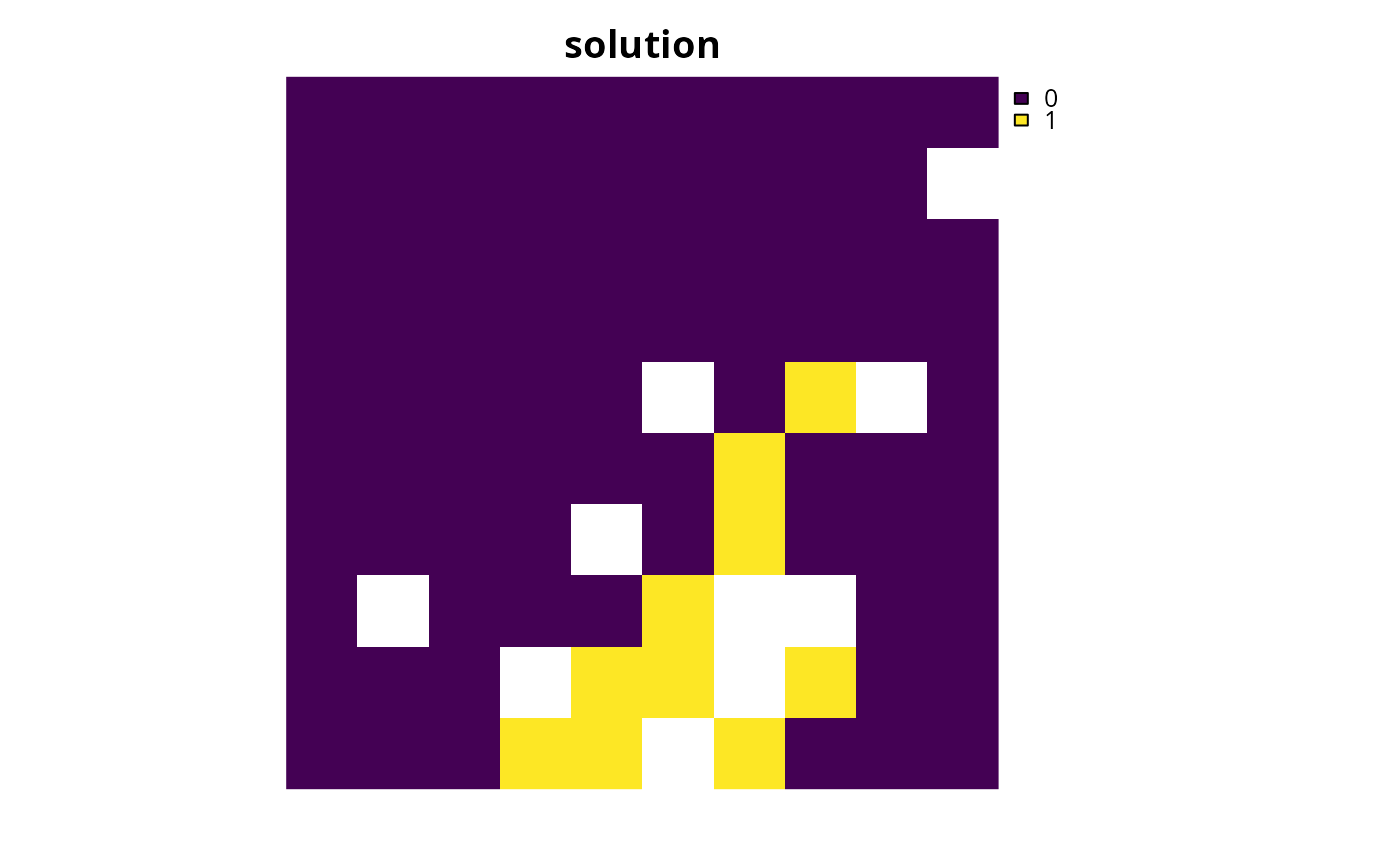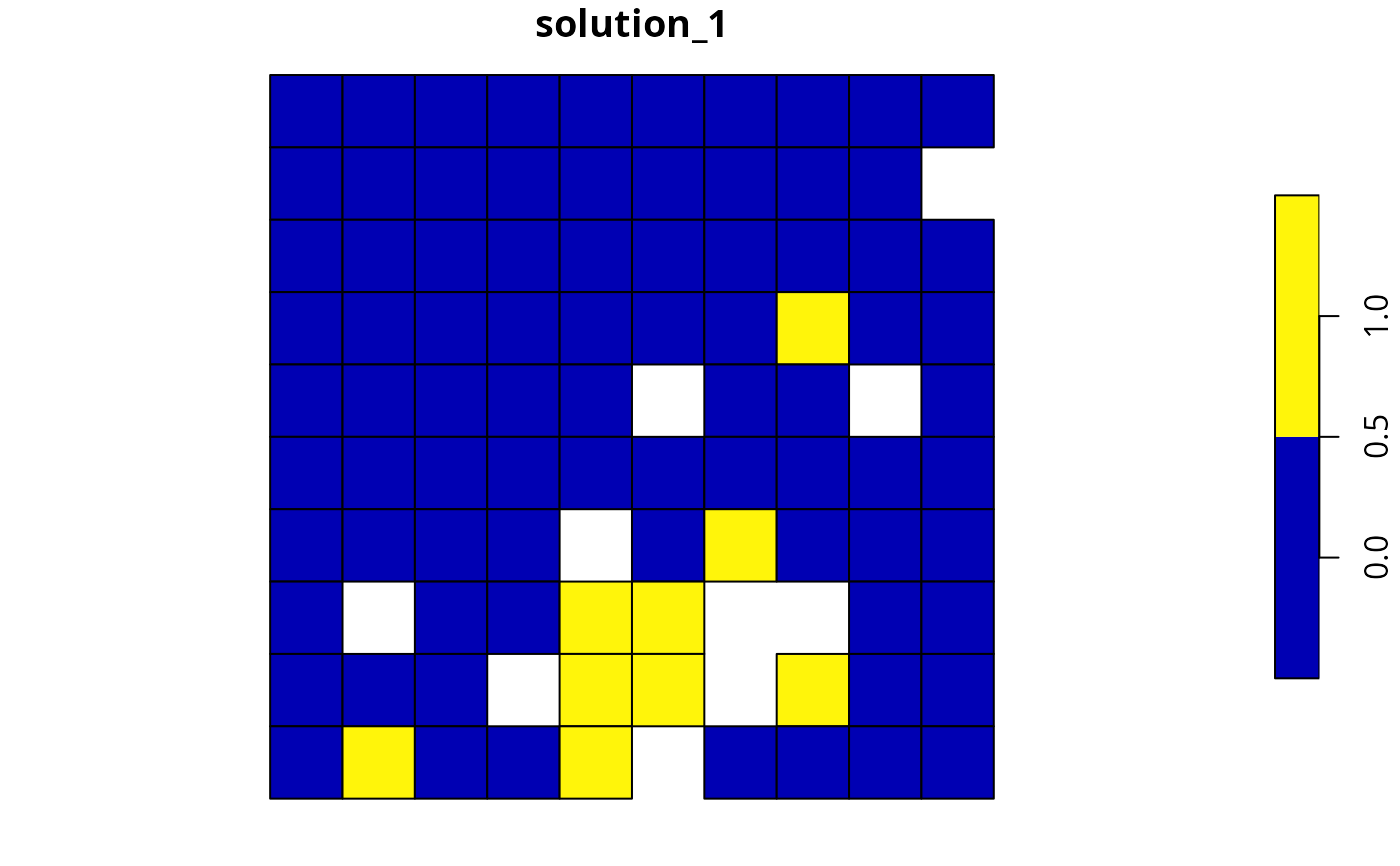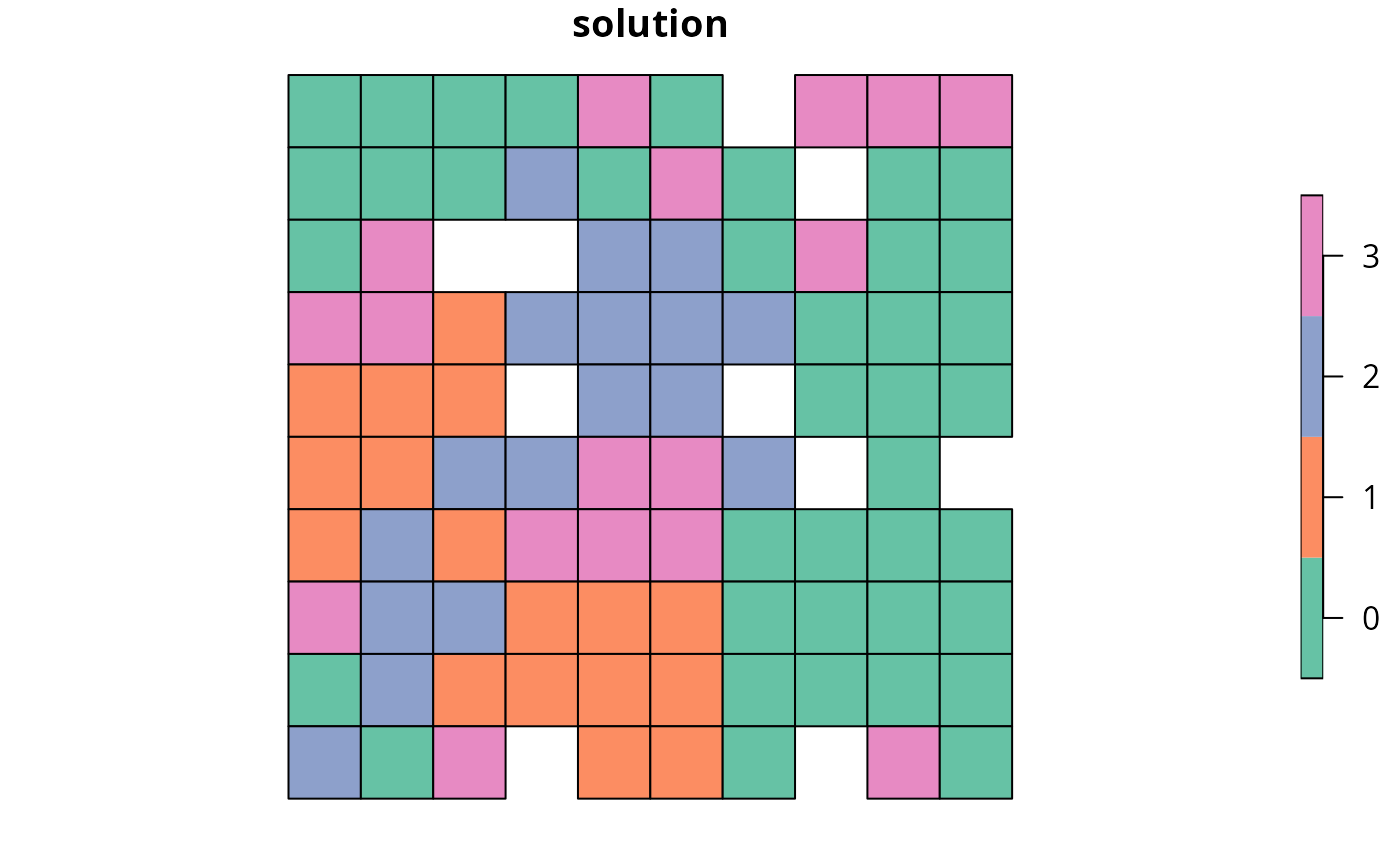Calculate the exposed boundary length (perimeter) associated with a solution to a conservation planning problem(). This summary statistic is useful for evaluating the spatial fragmentation of planning units selected within a solution.

eval_boundary_summary(x, ...)

# S3 method for default
eval_boundary_summary(x, ...)

# S3 method for ConservationProblem
eval_boundary_summary(
x,
solution,
edge_factor = rep(0.5, number_of_zones(x)),
zones = diag(number_of_zones(x)),
data = NULL,
...
)

## Arguments

x

problem() (i.e., ConservationProblem) object.

...

not used.

solution

numeric, matrix, data.frame, Raster, Spatial, or sf::sf() object. The argument should be in the same format as the planning unit cost data in the argument to x. See the Solution format section for more information.

edge_factor

numeric proportion to scale planning unit edges (borders) that do not have any neighboring planning units. For example, an edge factor of 0.5 is commonly used to avoid overly penalizing planning units along a coastline. Note that this argument must have an element for each zone in the argument to x.

zones

matrix or Matrix object describing the clumping scheme for different zones. Each row and column corresponds to a different zone in the argument to x, and cell values indicate the relative importance of clumping planning units that are allocated to a combination of zones. Cell values along the diagonal of the matrix represent the relative importance of clumping planning units that are allocated to the same zone. Cell values must range between 1 and -1, where negative values favor solutions that spread out planning units. The default argument to zones is an identity matrix (i.e., a matrix with ones along the matrix diagonal and zeros elsewhere), so that penalties are incurred when neighboring planning units are not assigned to the same zone. If the cells along the matrix diagonal contain markedly smaller values than those found elsewhere in the matrix, then solutions are preferred that surround planning units with those allocated to different zones (i.e., greater spatial fragmentation).

data

NULL, data.frame, matrix, or Matrix object containing the boundary data. These data describe the total amount of boundary (perimeter) length for each planning unit, and the amount of boundary (perimeter) length shared between different planning units (i.e., planning units that are adjacent to each other). See the Data format section for more information.

## Value

tibble::tibble() object containing the boundary length of the solution. It contains the following columns:

summary

character description of the summary statistic. The statistic associated with the "overall" value in this column is calculated using the entire solution (including all management zones if there are multiple zones). If multiple management zones are present, then summary statistics are also provided for each zone separately (indicated using zone names).

boundary

numeric exposed boundary length value. Greater values correspond to solutions with greater boundary length and, in turn, greater spatial fragmentation. Thus conservation planning exercises typically prefer solutions with smaller values.

## Details

This summary statistic is equivalent to the Connectivity_Edge metric reported by the Marxan software (Ball et al. 2009). It is calculated using the same equations used to penalize solutions according to their total exposed boundary (i.e., add_boundary_penalties()). See the Examples section for examples on how differences zone arguments can be used to calculate boundaries for different combinations of zones.

## Data format

The argument to data can be specified using the following formats. Note that boundary data must always describe symmetric relationships between planning units.

data as a NULL value

indicating that the data should be automatically calculated using the boundary_matrix() function. This argument is the default. Note that the boundary data must be supplied using one of the other formats below if the planning unit data in the argument to x do not explicitly contain spatial information (e.g., planning unit data are a data.frame or numeric class).

data as a matrix/Matrix object

where rows and columns represent different planning units and the value of each cell represents the amount of shared boundary length between two different planning units. Cells that occur along the matrix diagonal represent the amount of exposed boundary associated with each planning unit that has no neighbor (e.g., these value might pertain to boundaries along a coastline).

data as a data.frame object

with the columns "id1", "id2", and "boundary". The "id1" and "id2" columns contain identifiers (indices) for a pair of planning units, and the "boundary" column contains the amount of shared boundary length between these two planning units. This format follows the the standard Marxan format for boundary data (i.e., per the "bound.dat" file).

## Solution format

Broadly speaking, the argument to solution must be in the same format as the planning unit data in the argument to x. Further details on the correct format are listed separately for each of the different planning unit data formats:

x has numeric planning units

The argument to solution must be a numeric vector with each element corresponding to a different planning unit. It should have the same number of planning units as those in the argument to x. Additionally, any planning units missing cost (NA) values should also have missing (NA) values in the argument to solution.

x has matrix planning units

The argument to solution must be a matrix vector with each row corresponding to a different planning unit, and each column correspond to a different management zone. It should have the same number of planning units and zones as those in the argument to x. Additionally, any planning units missing cost (NA) values for a particular zone should also have a missing (NA) values in the argument to solution.

x has Raster planning units

The argument to solution be a Raster object where different grid cells (pixels) correspond to different planning units and layers correspond to a different management zones. It should have the same dimensionality (rows, columns, layers), resolution, extent, and coordinate reference system as the planning units in the argument to x. Additionally, any planning units missing cost (NA) values for a particular zone should also have missing (NA) values in the argument to solution.

x has data.frame planning units

The argument to solution must be a data.frame with each column corresponding to a different zone, each row corresponding to a different planning unit, and cell values corresponding to the solution value. This means that if a data.frame object containing the solution also contains additional columns, then these columns will need to be subsetted prior to using this function (see below for example with sf::sf() data). Additionally, any planning units missing cost (NA) values for a particular zone should also have missing (NA) values in the argument to solution.

x has Spatial planning units

The argument to solution must be a Spatial object with each column corresponding to a different zone, each row corresponding to a different planning unit, and cell values corresponding to the solution value. This means that if the Spatial object containing the solution also contains additional columns, then these columns will need to be subsetted prior to using this function (see below for example with sf::sf() data). Additionally, the argument to solution must also have the same coordinate reference system as the planning unit data. Furthermore, any planning units missing cost (NA) values for a particular zone should also have missing (NA) values in the argument to solution.

x has sf::sf() planning units

The argument to solution must be a sf::sf() object with each column corresponding to a different zone, each row corresponding to a different planning unit, and cell values corresponding to the solution value. This means that if the sf::sf() object containing the solution also contains additional columns, then these columns will need to be subsetted prior to using this function (see below for example). Additionally, the argument to solution must also have the same coordinate reference system as the planning unit data. Furthermore, any planning units missing cost (NA) values for a particular zone should also have missing (NA) values in the argument to solution.

See summaries for an overview of all functions for summarizing solutions. Also, see add_boundary_penalties() to penalize solutions with high boundary length.

Other summaries: eval_asym_connectivity_summary(), eval_connectivity_summary(), eval_cost_summary(), eval_feature_representation_summary(), eval_n_summary(), eval_target_coverage_summary()

## Examples

# \dontrun{
# set seed for reproducibility
set.seed(500)

data(sim_pu_raster, sim_pu_sf, sim_features,
sim_pu_zones_sf, sim_features_zones)

# build minimal conservation problem with raster data
p1 <- problem(sim_pu_raster, sim_features) %>%

# solve the problem
s1 <- solve(p1)

# print solution
print(s1)
#> class      : RasterLayer
#> dimensions : 10, 10, 100  (nrow, ncol, ncell)
#> resolution : 0.1, 0.1  (x, y)
#> extent     : 0, 1, 0, 1  (xmin, xmax, ymin, ymax)
#> crs        : NA
#> source     : memory
#> names      : layer
#> values     : 0, 1  (min, max)
#>

# plot solution
plot(s1, main = "solution", axes = FALSE, box = FALSE)# calculate boundary associated with the solution
r1 <- eval_boundary_summary(p1, s1)
print(r1)
#> # A tibble: 1 × 2
#>   summary boundary
#>   <chr>      <dbl>
#> 1 overall     2.25

# build minimal conservation problem with polygon (sf) data
p2 <- problem(sim_pu_sf, sim_features, cost_column = "cost") %>%

# solve the problem
s2 <- solve(p2)

# print first six rows of the attribute table
#> Simple feature collection with 6 features and 4 fields
#> Geometry type: POLYGON
#> Dimension:     XY
#> Bounding box:  xmin: 0 ymin: 0.9 xmax: 0.6 ymax: 1
#> CRS:           NA
#>       cost locked_in locked_out solution_1                       geometry
#> 1 215.8638     FALSE      FALSE          0 POLYGON ((0 1, 0.1 1, 0.1 0...
#> 2 212.7823     FALSE      FALSE          0 POLYGON ((0.1 1, 0.2 1, 0.2...
#> 3 207.4962     FALSE      FALSE          0 POLYGON ((0.2 1, 0.3 1, 0.3...
#> 4 208.9322     FALSE       TRUE          0 POLYGON ((0.3 1, 0.4 1, 0.4...
#> 5 214.0419     FALSE      FALSE          0 POLYGON ((0.4 1, 0.5 1, 0.5...
#> 6 213.7636     FALSE      FALSE          0 POLYGON ((0.5 1, 0.6 1, 0.6...

# plot solution
plot(s2[, "solution_1"])# calculate boundary associated with the solution
r2 <- eval_boundary_summary(p2, s2[, "solution_1"])
print(r2)
#> # A tibble: 1 × 2
#>   summary boundary
#>   <chr>      <dbl>
#> 1 overall     2.05

# build multi-zone conservation problem with polygon (sf) data
p3 <- problem(sim_pu_zones_sf, sim_features_zones,
cost_column = c("cost_1", "cost_2", "cost_3")) %>%
add_relative_targets(matrix(runif(15, 0.1, 0.2), nrow = 5,
ncol = 3)) %>%

# solve the problem
s3 <- solve(p3)

# print first six rows of the attribute table
#> Simple feature collection with 6 features and 9 fields
#> Geometry type: POLYGON
#> Dimension:     XY
#> Bounding box:  xmin: 0 ymin: 0.9 xmax: 0.6 ymax: 1
#> CRS:           NA
#>     cost_1   cost_2   cost_3 locked_1 locked_2 locked_3 solution_1_zone_1
#> 1 215.8638 183.3344 205.4113    FALSE    FALSE    FALSE                 0
#> 2 212.7823 189.4978 209.6404    FALSE    FALSE    FALSE                 0
#> 3 207.4962 193.6007 215.4212     TRUE    FALSE    FALSE                 0
#> 4 208.9322 197.5897 218.5241    FALSE    FALSE    FALSE                 0
#> 5 214.0419 199.8033 220.7100    FALSE    FALSE    FALSE                 0
#> 6 213.7636 203.1867 224.6809    FALSE    FALSE    FALSE                 0
#>   solution_1_zone_2 solution_1_zone_3                       geometry
#> 1                 0                 0 POLYGON ((0 1, 0.1 1, 0.1 0...
#> 2                 0                 1 POLYGON ((0.1 1, 0.2 1, 0.2...
#> 3                 0                 0 POLYGON ((0.2 1, 0.3 1, 0.3...
#> 4                 0                 1 POLYGON ((0.3 1, 0.4 1, 0.4...
#> 5                 0                 1 POLYGON ((0.4 1, 0.5 1, 0.5...
#> 6                 0                 0 POLYGON ((0.5 1, 0.6 1, 0.6...

# create new column representing the zone id that each planning unit
# was allocated to in the solution
s3$solution <- category_vector( s3[, c("solution_1_zone_1", "solution_1_zone_2", "solution_1_zone_3")]) s3$solution <- factor(s3\$solution)

# plot solution
plot(s3[, "solution"])# calculate boundary associated with the solution
# here we will use the default argument for zones which treats each
# zone as completely separate, meaning that the "overall"
# boundary is just the sum of the boundaries for each zone
r3 <- eval_boundary_summary(
p3, s3[, c("solution_1_zone_1", "solution_1_zone_2", "solution_1_zone_3")])
print(r3)
#> # A tibble: 4 × 2
#>   summary boundary
#>   <chr>      <dbl>
#> 1 overall     14.2
#> 2 zone_1       4.7
#> 3 zone_2       4.9
#> 4 zone_3       4.6

# let's calculate the overall exposed boundary across the entire
# solution, assuming that the shared boundaries between planning
# units allocated to different zones "count" just as much
# as those for planning units allocated to the same zone

# in other words, let's calculate the overall exposed boundary
# across the entire solution by "combining" all selected planning units
# together (regardless of which zone they are allocated to in the solution)
r3_combined <- eval_boundary_summary(
p3, zones = matrix(1, ncol = 3, nrow = 3),
s3[, c("solution_1_zone_1", "solution_1_zone_2", "solution_1_zone_3")])
print(r3_combined)
#> # A tibble: 4 × 2
#>   summary boundary
#>   <chr>      <dbl>
#> 1 overall     8.80
#> 2 zone_1      4.7
#> 3 zone_2      4.9
#> 4 zone_3      4.6

# we can see that the "overall" boundary is now less than the
# sum of the individual zone boundaries, because it does not
# consider the shared boundary between two planning units allocated to
# different zones as "exposed" when performing the calculations
# }